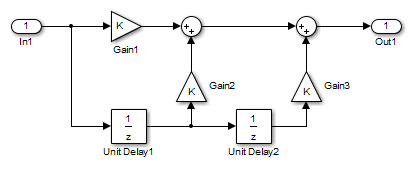Documentation

## Variable-Size Signal Basics

### About Variable-Size Signals

A Simulink® signal can be a scalar, vector (1-D), matrix (2-D), or N-D. For information about these types of signals, see Signal Basics in the Simulink User's Guide.

A Simulink variable-size signal is a signal whose size (the number of elements in a dimension), in addition to its values, can change during a model simulation. However, during a simulation, the number of dimensions cannot change. This capability allows you to model systems with varying resources, constraints, and environments.

### Creating Variable-Size Signals

You can create variable-size signals in your Simulink model by using:

• Switch or Multiport Switch blocks with different input ports having fixed-size signals with different sizes. The output is a variable-size signal.

• A selector block and the ```Starting and ending indices (port)``` indexing option. The index port signal can specify different subregions of the input data signal which produce an output signal of variable size as the simulation progresses.

• The S-function block with the output port configured for a variable-size signal. The output includes not only the values but also the dimension of the signal.

### How Variable-Size Signals Propagate

In the Simulink environment, variable-size signals can change their size during model execution in one of two ways:

• At every step of model execution.

Various blocks in the model modify the sizes of the signals during execution of the output method.

• Only during initialization of conditionally executed subsystems.

Size changes occur during distinct mode-switching events in subsystems such as Action, Enable, and Function-Call subsystems.

You can see the key difference by considering a Discrete 2-Tap Filter block with states.

Discrete 2-Tap FilterAssume that the input signal dimension to this filter changes from 4 to 1 during simulation. It is ambiguous when and how the states of the Unit Delay blocks should adapt from 4 to 1 to continue processing the input. To ensure consistency, both Unit Delay blocks must change their state behavior synchronously. To prevent ambiguity, Simulink generally disallows blocks whose number of states depends on input signal sizes in contexts where signal sizes change at any point during execution.

In contrast, consider the same Discrete 2-Tap Filter block in a Function-Call subsystem. Assume that this subsystem is using the second way to propagate variable-size signals. In this case, the size of the input signal changes from 4 to 1 only at the initialization of the subsystem. At initialization, the subsystem resets all of its states (including the states of the two Unit Delay blocks) to their initial values. Resetting the subsystem ensures no ambiguity on the assignment of states to the input signal of the filter.

Mode-Dependent Variable-Size Signals shows how you can use the two ways of propagating variable-size signals in a complementary fashion to model complex systems.

### Programmatically Determine Whether Signal Line Has Variable Size

This example shows how to use commands at the command prompt or in a script to determine whether a signal line has a variable size. In a large model or hierarchy of subsystems or referenced models, use this technique to determine whether a signal has a variable size due to an upstream block.

The example model `sldemo_varsize_basic` contains a signal `a` that is downstream of a Switch block. Use commands at the command prompt to determine whether `a` has a variable size.

1. Open the example model.

2. Select the Sum block whose output signal is labeled `a`.

3. At the command prompt, set the model to a compiled state (similar to a diagram update).

`sldemo_varsize_basic([],[],[],'compile')`

4. Get a handle to the block output port.

```portHandles = get_param(gcb,'portHandles'); outPortHandle = portHandles.Outport;```

5. Query the programmatic parameter `CompiledPortDimensionsMode` of the output port.

`varSize = get_param(outPortHandle,'CompiledPortDimensionsMode')`
```varSize = 1```

The value of the variable `varSize` is `1`, which indicates that the signal `a` has variable size.

The value `0` indicates that a signal does not have variable size.

6. Terminate the model compilation.

`sldemo_varsize_basic([],[],[],'term')`

### Empty Signals

An empty signal is a signal with a length of `0`. For example, signals with size ``, `[0x3]`, `[2x0]`, and `[2x0x3]` are all empty signals. Simulink allows empty signals with variable-size signals and supports most element-wise operations. However, Simulink does not support empty signals for blocks that modify signal dimensions. Unsupported blocks include Reshape, Permute, and Sum along a specified dimension.

### Subsystem Initialization of Variable-Size Signals

The initial signal size from an Outport block in a conditionally executed subsystem varies depending on the parameters you select.

If you set the Propagate sizes of variable-size signals parameter in the parent subsystem to `During execution`, the Initial output parameter for the Output block must not exceed the maximum size of the input port. If the Initial output parameter value is:

Initial output parameterInitial output signal size
A nonscalar matrixThe initial output signal size is the size of the Initial output parameter.
A scalarThe initial output signal size is a scalar.
The default []The initial output size is an empty signal (dimensions are all zeros).

If you set the Propagate sizes of variable-size signals parameter in the parent subsystem to `Only when enabling`, the Initial output parameter for the Output block must be a scalar value.

• When size is repropagated for the input of the Outport block, the initial output value is set using scalar expansion from the scalar parameter value.

• If the Initial output parameter is the default value `[]`, Simulink treats the initial output as a grounded value.

• If the model does not activate the parent subsystem at start time (```t = 0```), the current size of the subsystem output corresponding to the Outport block is set to maximum size.

• When its parent subsystem repropagates signal sizes, the values of the subsystem variable-size output signals are also reset to their initial output parameter values.